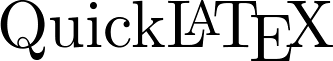Advanced LaTeX plugin. Copy-paste exchange with offline papers. Allows custom preamble/TexLive packages. Supports TikZ, proper formulae alignment.

Standard LaTeX expressions can be cut and pasted directly into WordPress posts, pages, and comments; display environments require no enclosures, other expressions require only a surrounding[latexpage]

At first, we sample $f(x)$ in the $N$ ($N$ is odd) equidistant points around $x^*$:
$f_k = f(x_k),\: x_k = x^*+kh,\: k=-\frac{N-1}{2},\dots,\frac{N-1}{2}$
where $h$ is some step.
Then we interpolate points $\{(x_k,f_k)\}$ by polynomial
\begin{equation} \label{eq:poly}
P_{N-1}(x)=\sum_{j=0}^{N-1}{a_jx^j}
\end{equation}
Its coefficients $\{a_j\}$ are found as a solution of system of linear equations:
\begin{equation} \label{eq:sys}
\end{equation}
Here are references to existing equations: (eq:poly), (eq:sys).
Here is reference to non-existing equation (eq:unknown).

[toggle Title=”查看使用截图”]

LaTeX – enabled post in WordPress editor.Same post – published.TikZ drawing inclusion (up: source code in the post editor, down: published post).Admin page – LaTeX Syntax Sitewide & Custom preambleAdmin page – Image format and other system settings.

Debug Mode – Error message triggered by misspelled sqrt command.[/toggle]